JEE  >  JEE Main Previous year questions (2021-22): Properties of Matter - 2

# JEE Main Previous year questions (2021-22): Properties of Matter - 2 - Notes | Study Physics 35 Years JEE Main & Advanced Past year Papers - JEE

 1 Crore+ students have signed up on EduRev. Have you?

Q.41. A water drop of radius 1 μm falls in a situation where the effect of buoyant force is negligible. Co-efficient of viscosity of air is 1.8 × 10−5 Nsm−2 and its density is negligible as compared to that of water 106 gm−3. Terminal velocity of the water drop is :
(Take acceleration due to gravity = 10 ms−2)    (JEE Main 2022)
(a) 145.4 × 10−6 ms−1
(b) 118.0 × 10−6 ms−1
(c) 132.6 × 10−6 ms−1
(d) 123.4 × 10−6 ms−1

Ans. d
6πηrv = mg
6πηrv = 4/3πr3ρg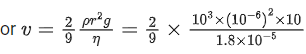=123.4 x 10−6 m/s

Q.42. A wire of length L is hanging from a fixed support. The length changes to L1 and L2 when masses 1 kg and 2 kg are suspended respectively from its free end. Then the value of L is equal to:    (JEE Main 2022)
(a)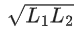(b)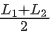(c) 2L1−L2
(d) 3L1−2L2

Ans. c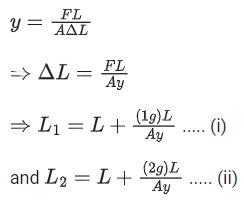⇒ L = 2L1 − L2

Q.43. A cylindrical tube, with its base as shown in the figure, is filled with water. It is moving down with a constant acceleration a along a fixed inclined plane with angle θ = 45. P1 and P2 are pressures at points 1 and 2, respectively, located at the base of the tube. Let β = (P1 − P2)/(ρgd), where ρ is density of water, d is the inner diameter of the tube and g is the acceleration due to gravity. Which of the following statement(s) is(are) correct?    (JEE Advanced 2021)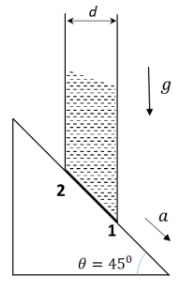(a) β = 0 when a = g/√2
(b) β > 0 when a = g/√2
(c) β = √2−1/√2 when a = g/2
(d) β = 1/√2 when a = g/2

Ans. a, c
(p1−p2)ds = ρdsd√2(g sin⁡45 − a)
(p1−p2)ds = ρd(g−a√2)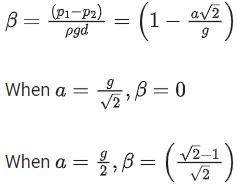Q.44. A bob of mass 'm' suspended by a thread of length l undergoes simple harmonic oscillations with time period T. If the bob is immersed in a liquid that has density 1/4 times that of the bob and the length of the thread is increased by 1/3rd of the original length, then the time period of the simple harmonic oscillations will be :-    (JEE Main 2021)
(a) T
(b) 32T
(c) 34T
(d) 43T

Ans. d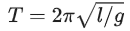When bob is immersed in liquid
mgeff = mg − Buoyant force
mgeff = mg − vσg (σ = density of liquid)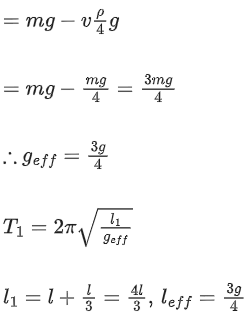By solving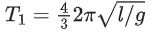T= 4T/3

Q.44. Four identical hollow cylindrical columns of mild steel support a big structure of mass 50 × 103 kg. The inner and outer radii of each column are 50 cm and 100 cm respectively. Assuming uniform local distribution, calculate the compression strain of each column. [Use Y = 2.0 × 1011 Pa, g = 9.8 m/s2]    (JEE Main 2021)
(a) 3.60 × 10−8
(b) 2.60 × 107
(c) 1.87 × 10−3
(d) 7.07 × 10−4

Ans. b
Force on each column = mg/4
Strain = mg/4AY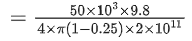= 2.6 × 10−7

Q.45. A uniform heavy rod of weight 10 kg ms−2, cross-sectional area 100 cmand length 20 cm is hanging from a fixed support. Young modulus of the material of the rod is 2 × 1011 Nm−2. Neglecting the lateral contraction, find the elongation of rod due to its own weight.    (JEE Main 2021)
(a) 2 × 10−9 m
(b) 5 × 10−8 m
(c) 4 × 10−8 m
(d) 5 × 10−10 m

Ans. d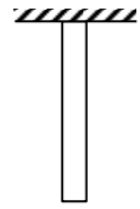We know,
Δl = WL/2AY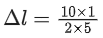× 100 × 10−4 × 2 × 1011
Δl = 1/2 × 10−9 = 5 × 10−10 m
Option (d)

Q.46. In Millikan's oil drop experiment, what is viscous force acting on an uncharged drop of radius 2.0 × 10−5 m and density 1.2 × 103 kgm−3 ? Take viscosity of liquid = 1.8 × 10−5 Nsm−2.(Neglect buoyancy due to air).    (JEE Main 2021)
(a) 3.8 × 10−11 N
(b) 3.9 × 10−10 N
(c) 1.8 × 10−10 N
(d) 5.8 × 10−10 N

Ans. b
Viscous force = Weight
= ρ × (4/3πr3)g
= 3.9 × 10−10

Q.47. Two blocks of masses 3 kg and 5 kg are connected by a metal wire going over a smooth pulley. The breaking stress of the metal is 24/π × 102 Nm-2. What is the minimum radius of the wire ? (Take g = 10 ms-2)    (JEE Main 2021)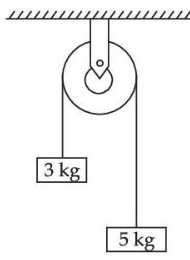(a) 125 cm
(b) 1250 cm
(c) 12.5 cm
(d) 1.25 cm

Ans. c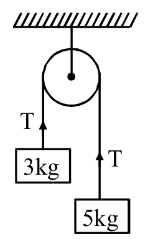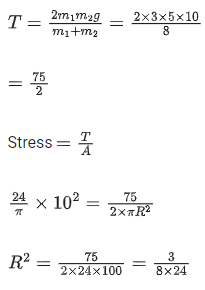⇒ R = 0.125 m
R = 12.5 cm

Q.48. Two narrow bores of diameter 5.0 mm and 8.0 mm are joined together to form a U-shaped tube open at both ends. If this U-tube contains water, what is the difference in the level of two limbs of the tube. [Take surface tension of water T = 7.3 × 10−2 Nm−1, angle of contact = 0, g = 10 ms2 and density of water = 1.0 × 103 kg m−3]    (JEE Main 2021)
(a) 3.62 mm
(b) 2.19 mm
(c) 5.34 mm
(d) 4.97 mm

Ans. b
We have, PA = PB. [Points A & B at same horizontal level]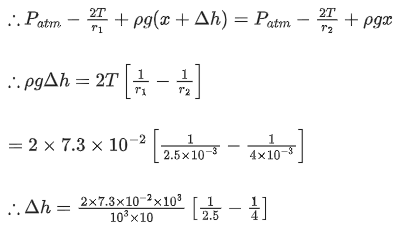= 2.19 × 10−3 m = 2.19 mm
Hence, option (b).

Q.49. A raindrop with radius R = 0.2 mm falls from a cloud at a height h = 2000 m above the ground. Assume that the drop is spherical throughout its fall and the force of buoyance may be neglected, then the terminal speed attained by the raindrop is :
[Density of water fw = 1000 kg m−3 and Density of air fa = 1.2 kg m−3, g = 10 m/s2, Coefficient of viscosity of air = 1.8 × 10−5 Nsm−2]    (JEE Main 2021)
(a) 250.6 ms−1
(b) 43.56 ms−1

(c) 4.94 ms−1
(d) 14.4 ms−1

Ans. c
At terminal speed
a = 0
Fnet = 0
mg = Fv = 6π ηRv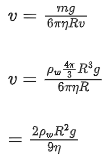= 400/81 m/s
= 4.94 m/s

Q.50. A light cylindrical vessel is kept on a horizontal surface. Area of base is A. A hole of cross-sectional area 'a' is made just at its bottom side. The minimum coefficient of friction necessary to prevent sliding the vessel due to the impact force of the emerging liquid is (a < < A) :    (JEE Main 2021)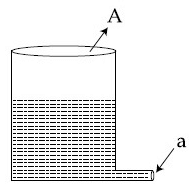(a) A/2a
(b) None of these
(c) 2a/A
(d) a/A

Ans. c
For no sliding
f ≥ ρav2
μmg ≥ ρav2
μρAhg ≥ ρa2gh
μ ≥ 2a/A
Option (3)

Q.51. Two series of same length and radius are joined end to end and loaded. The Young's modulii of the materials of the two wires are Y1 and Y2. The combination behaves as a single wire then its Young's modulus is :    (JEE Main 2021)
(a)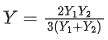(b)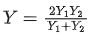(c)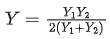(d)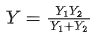Ans. b
In series combination Δl = l1 + l2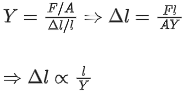Equivalent length of rod after joining is = 2l
As, lengths are same and force is also same in series
Δl = Δl1 + Δl2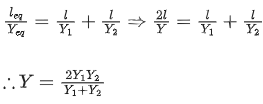Q.52. Two small drops of mercury each of radius R coalesce to form a single large drop. The ratio of total surface energy before and after the change is :    (JEE Main 2021)
(a) 21/3:1
(b) 1:21/3
(c) 2 : 1
(d) 1 : 2

Ans. a
Let r be radius of common drop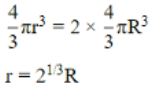surface tension of 2 drops 2 × 4πR2T
surface tension of common drop 4πr2T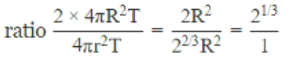Q.53. The value of tension in a long thin metal wire has been changed from T1 to T2. The lengths of the metal wire at two different values of tension T1 and T2 are l1 and l2 respectively. The actual length of the metal wire is :    (JEE Main 2021)
(a)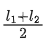(b)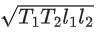(c)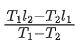(d)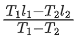Ans. c
Suppose, I0 be the actual length of metal wire and Y be its Young's modulus.
From Hooke's law,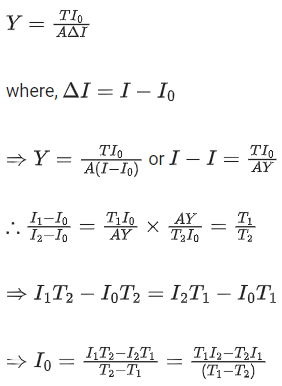Q.54.  An object is located at 2 km beneath the surface of the water. If the fractional compression ΔV/V is 1.36%, the ratio of hydraulic stress to the corresponding hydraulic strain will be ____________. [Given : density of water is 1000 kgm−3 and g = 9.8 ms−2]    (JEE Main 2021)
(a) 1.44 × 107 Nm−2
(b) 1.44 × 109 Nm−2
(c) 1.96 × 107 Nm−2
(d) 2.26 × 109 Nm−2

Ans. b⇒ β = 1.44 × 109 N/m2

Q.55. When two soap bubbles of radii a and b (b > a) coalesce, the radius of curvature of common surface is :    (JEE Main 2021)
(a)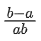(b)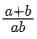(c)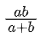(d)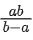Ans. d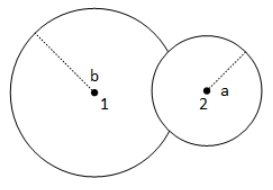P− P0 = 4S/b ....(1)
P2 − P0 − 4S/a .....(2)
P2−P= 4S/R ......(3)
eq(2) - eq(1) = eq(3)
⇒ 1/a − 1/b = 1/R
∴ R = ab/b−a

Q.56. What will be the nature of flow of water from a circular tap, when its flow rate increased from 0.18 L/min to 0.48 L/min? The radius of the tap and viscosity of water are 0.5 cm and 10−3 Pa s, respectively. (Density of water : 103 kg/m3)    (JEE Main 2021)
(c) Remains turbulent flow

Ans. a
The nature of flow is determined by reynolds no
R = ρVD/η
If R < 1000 → flow is steady
1000 < R < 2000 → flow becomes unsteady
R > 2000 → flow is turbulent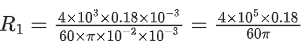= 0.0038 × 105 = 380
R= 0.48/0.18 × 380 = 1018

Q.57. The pressure acting on a submarine is 3 × 105 Pa at a certain depth. If the depth is doubled, the percentage increase in the pressure acting on the submarine would be :
(Assume that atmospheric pressure is 1 × 105 Pa density of water is 103 kg m−3, g = 10 ms−2)    (JEE Main 2021)
(a) 200/5%
(b) 200/3%
(c) 3/200%
(d) 5/200%

Ans. b
P = P0 + hρg = 3 × 105 Pa
⇒ hρg = 3 × 105 − 1 × 105
⇒ hρg = 2 × 105
∴ 2hρg = 4 × 105
∴ P' = P0 + 4 × 105
∴ P' = 5 × 105 Pa
∴ % increase in pressure = P′−P/P × 100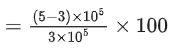=200/3%

Q.58. The length of metallic wire is l1 when tension in it is T1. It is l2 when the tension is T2. The original length of the wire will be :    (JEE Main 2021)
(a)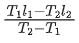(b)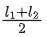(c)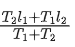(d)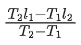Ans. d
Assuming Hooke's law to be valid.
T ∝ (Δl)
T = k(Δl)
Let, l0 = natural length (original length)
⇒T = k(l−l0)
so, T= k(l1−l0) & T= k(l2−l0)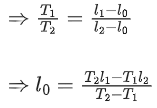Q.59. The normal density of a material is ρ and its bulk modulus of elasticity is K. The magnitude of increase in density of material, when a pressure P is applied uniformly on all sides, will be :    (JEE Main 2021)
(a) ρK/P
(b) PK/ρ
(c) ρP/K
(d) K/ρP

Ans. c
Bulk modulus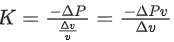We know, ρ = M/V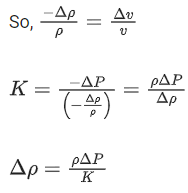Δρ = ρP/K

Q.60. A large number of water drops, each of radius r, combine to have a drop of radius R. If the surface tension is T and mechanical equivalent of heat is J, the rise in heat energy per unit volume will be :    (JEE Main 2021)
(a)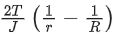(b)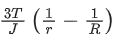(c) 3T/rJ
(d) 2T/rJ

Ans. b
R is the radius of bigger drop.
r is the radius of n water drops.
Water drops are combined to make bigger drop.
So,
Volume of n drops = volume of bigger drop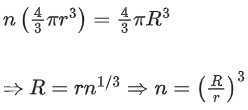Loss in surface energy, ΔU = T × (Change in surface area)
ΔU = T (n4πr2 − 4πR2)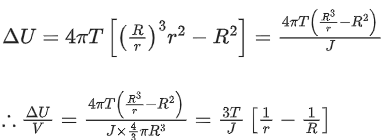Q.61. If Y, K and η are the values of Young's modulus, bulk modulus and modulus of rigidity of any material respectively. Choose the correct relation for these parameters.    (JEE Main 2021)
(a)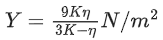(b)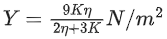(c)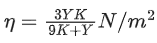(d)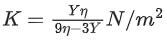Ans. d
We know that,
Y = 3K(1−2σ)
⇒ σ = 1/2(1 − Y3K) ..... (i)
Also, Y = 2η(1 + σ)
⇒ σ = Y/2η − 1 .... (ii)
On comparing Eqs. (i) and (ii), we get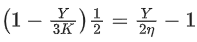On solving, we get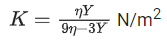Q.62. A steel rod with y = 2.0 × 1011 Nm−2 and α = 10−5 C−1 of length 4 m and area of cross-section 10 cm2 is heated from 0C to 400C without being allowed to extend. The tension produced in the rod is x × 105 N where the value of x is ____________.    (JEE Main 2021)

Ans. 8
Given, the Young's modulus of the steel rod, Y = 2 × 1011 Pa
Thermal coefficient of the steel rod, α = 10−5C
The length of the steel rod, l = 4 m
The area of the cross-section, A = 10 cm2
The temperature difference, ΔT = 400C
As we know that,
Thermal strain = α ΔT
Using the Hooke's law
Young's modulus (Y) = Thermalstress/Thermalstrain = F/AαΔT
Thermal stress, F = YAαΔT
Substitute the values in the above equation, we get
F = 2 × 1011 × 10 × 10−4 × 10−5 × (400)
= 8 × 105N
Comparing with, F = x × 105N
The value of the x = 8.

Q.63. When a rubber ball is taken to a depth of __________m in deep sea, its volume decreases by 0.5%. (The bulk modulus of rubber = 9.8 × 108 Nm−2, Density of sea water = 103 kgm−3, g = 9.8 m/s2)    (JEE Main 2021)

Ans. 500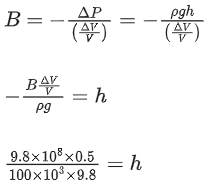h = 500

Q.64. Wires W1 and W2 are made of same material having the breaking stress of 1.25 × 109 N/m2. W1 and W2 have cross-sectional area of 8 × 10−7 m2 and 4 × 10−7 m2, respectively. Masses of 20 kg and 10 kg hang from them as shown in the figure. The maximum mass that can be placed in the pan without breaking the wires is ____________ kg. (Use g = 10 m/s2)    (JEE Main 2021)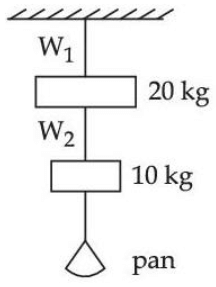Ans. 40

B.S1 = T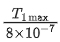⇒ T1 max = 8 × 1.25 × 100 = 1000 N
B.S2 =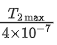⇒ T2 max = 4 × 1.25 × 100 = 500 N
m = 500−100/10 = 40 kg

Q.65. A soap bubble of radius 3 cm is formed inside the another soap bubble of radius 6 cm. The radius of an equivalent soap bubble which has the same excess pressure as inside the smaller bubble with respect to the atmospheric pressure is ________ cm.    (JEE Main 2021)

Ans. 2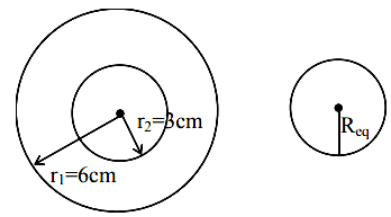Excess pressure inside the smaller soap bubble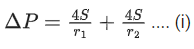The excess pressure inside equivalent soap bubble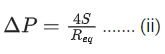From (i) & (ii)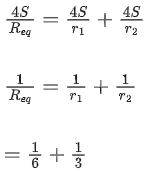Req = 2 cm

Q.66. The water is filled upto height of 12 m in a tank having vertical sidewalls. A hole is made in one of the walls at a depth 'h' below the water level. The value of 'h' for which the emerging steam of water strikes the ground at the maximum range is ________ m.    (JEE Main 2021)

Ans. 6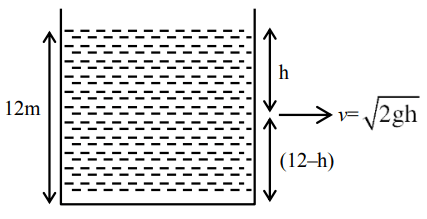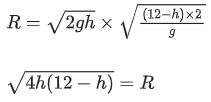For maximum R
dR/dh = 0
⇒ h = 6 m

Q.67. A stone of mass 20 g is projected from a rubber catapult of length 0.1 m and area of cross section 10−6 mstretched by an amount 0.04 m. The velocity of the projected stone is ______________ m/s.
(Young's modulus of rubber = 0.5 × 109 N/m2)    (JEE Main 2021)

Ans. 20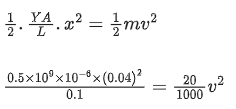∴ v= 400
v = 20 m/s

Q.68. The area of cross-section of a railway track is 0.01 m2. The temperature variation is 10C. Coefficient of liner expansion of material of track is 10−5/C. The energy stored per meter in the track is ____________ J/m.
(Young's modulus of material of track is 1011 Nm−2)    (JEE Main 2021)

Ans. 05

As the tracks won't be allowed to expand linearly, the rise in temperature would lead to developing thermal stress in track.
(Stress)/y = αΔT or σ = YαΔT
Energy stored per unit volume = 1/2 σ/Y
⇒ Energy stored per unit length = Aσ2/2Y
= A/2 × Yα2ΔT2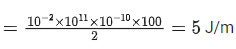Q.69. Consider a water tank as shown in the figure. It's cross-sectional area is 0.4 m2. The tank has an opening B near the bottom whose cross-section area is 1 cm2. A load of 24 kg is applied on the water at the top when the height of the water level is 40 cm above the bottom, the velocity of water coming out the opening B is v ms-1.
The value of v, to the nearest integer, is ___________. [Take value of g to be 10 ms-2]    (JEE Main 2021)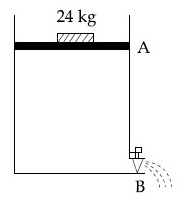Ans. 3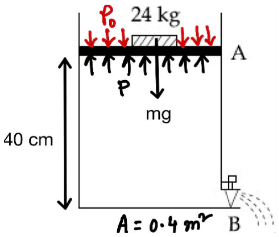Force at point A,
mg + p0A = pA
⇒ p = p0 + mgA
Given, area of A = 0.4 m2 = 0.4 × 104 cm2 and area of B = 1 cm2
applying continuity equation
AV= av [V1 = velocity at point A]
⇒ V1 = α/A v
As A >>> a so α/A very small
∴ V1 <<< v
So we can neglect V1 by assuming V= 0
On applying Bernoulli's theorem at points A and B,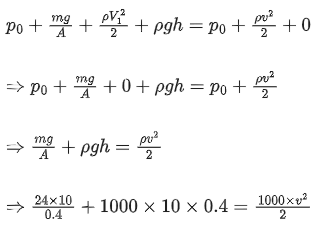⇒ v ≃ 3 m/s

Q.70. Suppose you have taken a dilute solution of oleic acid in such a way that its concentration becomes 0.01 cm3 of oleic acid per cm3 of the solution. Then you make a thin film of this solution (monomolecular thickness) of area 4 cm2 by considering 100 spherical drops of radius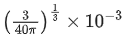cm. Then the thickness of oleic acid layer will be x × 10−14 m. Where x is ____________.    (JEE Main 2021)

Ans. 25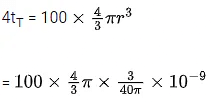= 10-8 cm3
⇒ tT = 25 × 10-10 cm
= 25 × 10-12 m
t0 = 0.01 tT = 25 × 10-14 m
∴ x = 25

Q.71. A hydraulic press can lift 100 kg when a mass 'm' is placed on the smaller piston. It can lift ___________ kg when the diameter of the larger piston is increased by 4 times and that of the smaller piston is decreased by 4 times keeping the same mass 'm' on the smaller piston.    (JEE Main 2021)

Ans. 25600
According to Pascal's law,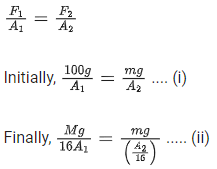On dividing Eqs. (i) by (ii), we get
(100 x 16)/M = 1/16
∴ M = 25600 kg

The document JEE Main Previous year questions (2021-22): Properties of Matter - 2 - Notes | Study Physics 35 Years JEE Main & Advanced Past year Papers - JEE is a part of the JEE Course Physics 35 Years JEE Main & Advanced Past year Papers.
All you need of JEE at this link: JEE

## Physics 35 Years JEE Main & Advanced Past year Papers

110 docs|49 tests
 Use Code STAYHOME200 and get INR 200 additional OFF

## Physics 35 Years JEE Main & Advanced Past year Papers

110 docs|49 tests

Track your progress, build streaks, highlight & save important lessons and more!

,

,

,

,

,

,

,

,

,

,

,

,

,

,

,

,

,

,

,

,

,

;